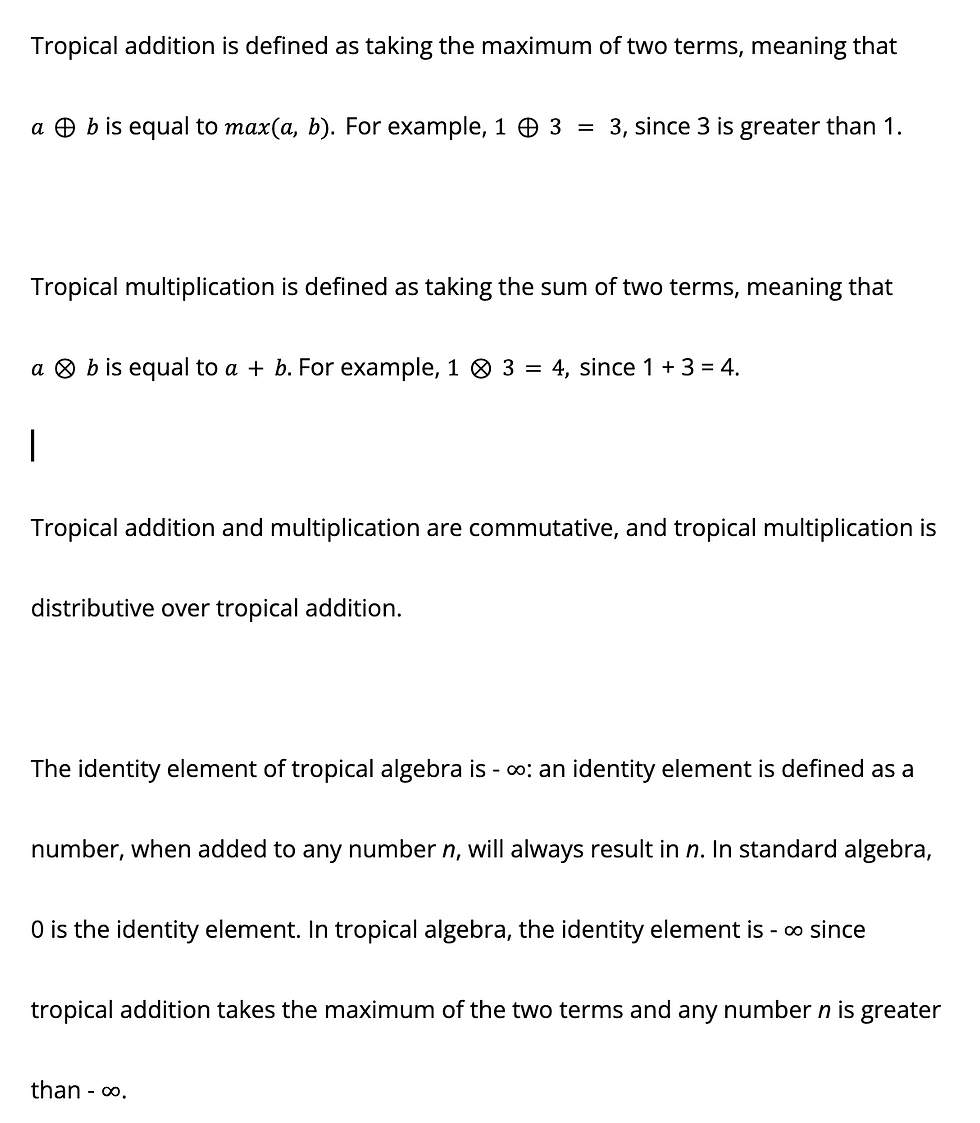top of page
Search

# What is Tropical Algebra?

Updated: Jan 7(Cover Artist: Sophie Cheng)

Introduction

Tropical algebra is a relatively new form of algebra used in tropical geometry. This field was pioneered by Imre Simon, a computer scientist who pioneered the field and traveled across the Tropic of Capricorn. Tropical algebra and tropical geometry was further developed by a conglomerate of individual mathematicians, including Victor Maslov and Grigory Mikhalkin. Tropical geometry initially only held applications in discrete mathematics, but now also has powerful applications in algebraic geometry and combinatorics.

Tropical algebra is used in tropical geometry, which can often simplify complex equations. In tropical algebra, standard arithmetic polynomials and graphs are completely redefined. Although tropical geometry is still a new area of research, tropical geometry is significant as it has many applications in other areas of math, such as combinatorics and complex geometry.

Tropical Operations

The operations and notations of tropical geometry are very different from those of standard algebra, or “regular” algebra. While there is some discrepancy in the notation of tropical algebra, in general, tropical addition is notated with:Tropical multiplication is notated with:Tropical equations and expressions can also be written as standard algebra, with quotations surrounding the equations and expressions.A multiplicative identity is defined as a number, when multiplied by any number n, will always result in n: the multiplicative identity of tropical algebra is 0. In standard algebra, 1 is the multiplicative identity. In tropical algebra, the multiplicative identity is 0 since tropical multiplication takes the sum of two terms -- for any number n and 0, n will always be the sum of the two terms.

Tropical Polynomials

In standard algebra, polynomials in standard form are written as:Since addition and multiplication are defined differently in tropical algebra, tropical polynomials will take on a different form. In tropical algebra, polynomials in standard form are written as:Note that in tropical algebra, exponents are written in a different way than they are written in standard algebra. For example, x^n in standard algebra would be rewritten as nx, since x^n can be expanded to "x times x", n times. In tropical algebra, multiplication is defined as standard addition, so x^n is written as nx in tropical algebra.

The fundamental theorem of standard algebra also applies to tropical algebra: given a tropical polynomial of degree n, there will be n roots.

Graphing

When graphed, tropical polynomials are linear and piecewise. The roots of tropical polynomials are shown in the graph as the "corners," or vertices, of the graph. Roots of polynomials are defined differently in tropical algebra - the roots of a tropical polynomial are values of x where a maximum is achieved twice. This means that given a value of x, there will be two terms that are the maximum. The only way this is possible is if these terms are equivalent and the maximum value. Two terms must be the maximum because a polynomial in tropical form breaks down the terms of the polynomial in standard form, so that the polynomial looks for the maximum of a set of terms.

For example, the standard polynomial P(x) = x2+2x+1 is rewritten as max(2x, x+2, 1) in tropical form. This comes from converting the addition and multiplication to tropical algebra. In addition, we take the maximum of the three terms, as addition is taking the maximum in tropical algebra - so \$P(x) = max[(x^{2}), (2x), 1]\$. Within the terms, with multiplication, we convert this to addition in tropical algebra - so we're left with \$P(x) = max [2x, x+2, 1]\$. The roots would be any value of x where two terms are the maximum.

For 2x = x+2, x = 2, which is the maximum value for the three terms, so x = 2 is a root. For 2x = 1, x = ½, which is not the maximum value for the three terms, so x = 1/2 is not a root. For x+2 = 1, x = -1, which is not the maximum value for the three terms, so x = -1 is not a root.

In another example, the standard polynomial P(x) = x + 0 is rewritten as max ( 0, x) in tropical form. The root for this polynomial is x = 0, where both terms are at the maximum value of 0. The graph for this tropical polynomial is shown below:Note the “corner” of the graph is formed at the root, x = 0, where both rays of the graph intersect at the point (0, 0). Note the two rays that make up this graph are y = 0 for x ≤ 0 and y = x for x ≥ 0. To the right of the point (0, 0), only the term x is the maximum, which doesn’t satisfy the conditions to be a root. To the right of the point (0, 0), only the term 0 is the maximum as x decreases, which also doesn’t satisfy the conditions.

This article was a brief discussion of the foundations of tropical algebra, covering its history, operations and graphing. Tropical algebra is a rather new topic - for this reason, much more research has to be done, and much more standardized notation has to be composed. However, despite its novelty, this topic truly holds many applications, the interesting of which include tropical geometry, the study of phylogenetic trees, and computer science.